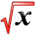# First and Second Derivative TestsThis article is part of the MathHelp Tutoring Wiki

First Derivative Test If there is a critical number "c" for a continuous function, then 1) if f' changes from (+) to (-) at c, f has a local maximum at c. 2) If f' changes from (-) to (+) at c, then f has a local minimum at c. 3) If f' does not change sign at c, then f has no local maximum or minimum.

Second Derivative Test If f is continuous by c, the 1) if the f'(c) is greater than zero, f has a local minimum at c 2) if f'(c)=zero and f(c) is less than zero, f has a local maximum at c.

Inflection point: A point on the graph where the curve changes from concave up to concave down. Concavity Test: If f is greater than zero, f is concave up, if f is less than zero, f is concave down

EXAMPLE: Sketch the function y=x4 - 4x3.

Step 1. Write the f' and f

Step 2. Find the critical points by setting f'=0

Step 3. Evaluate f" at critical points

Step 4. Determine the concavity:

If f is <0, it is concave down

If f is >0, it is concave up

A point where the concavity changes from up to down or vice-versa is called an inflection point

EXAMPLE 2: For the function, f(x)=4x3-2x2+1, find where the function is increasing/decreasing and the concavity.

Solution:

1. f'= 12x2-4x , f"= 24x-4

2. Set f'=0 --> 12x2-4x =0

3x2-4x =0

x(3x-4)=0

x=0, x=4/3

3. Evaluate the f" at the critical points

f"(0)=24(0)-4= -4

f"(4/3)=24(4/3)-4= 28

4. Concavity